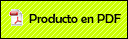# MATHEMATICAL THINKING, CONCEPTUAL FRAMEWORKS: A REVIEW OF STRUCTURES FOR ANALYZING PROBLEM-SOLVING PROTOCOLS

###Barrera Mora, Fernando2010

Fernando Hitt, Fernando Barrera Mora and Matías Camacho Machín (2010). Mathematical thinking, conceptual frameworks: A review of structures for analyzing problem-solving protocols. Far East Journal of Mathematical Education Volume 4(2). Preprinted

Abstract

Recently, it has been considered important to reflect on the coincidences between the mathematical thinking of the School of Mathematics and the Discipline of Mathematics. It is widely accepted that a professional mathematician has naturally developed reasoning abilities that are essential to his practice. Such reasoning abilities are considered central to student learning at different educational levels. While the problem is not entirelynew, the enrollment diminished in science and engineering university programs, suggests new reflections that might help the community when proposing scientific and engineering training programs. In this paper, we conduct an analysis of the processes developed by a mathematician during problem solving activities, with the aim of identifying relevant characteristics of the structure representations of mathematical concepts that he shows to solve problems. In the same lines, we pose the question: how this structure influences the mathematical creativity? . The mathematics teacher, by knowing the characteristics of mathematical reasoning and the limitations to promoting them in the classroom, is better positioned to help his students in their learning processes.Resolución de problemas y uso de tecnologías digitales en el desarrollo de competencias matemáticas

Mathematical thinking, conceptual frameworks: a review of structures for analyzing problema- solving...

MATHEMATICAL THINKING, CONCEPTUAL FRAMEWORKS: A REVIEW OF STRUCTURES FOR ANALYZING PROBLEM-SOLVING P...